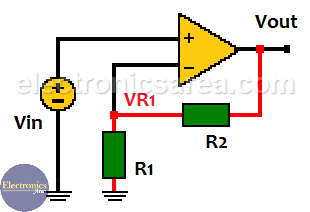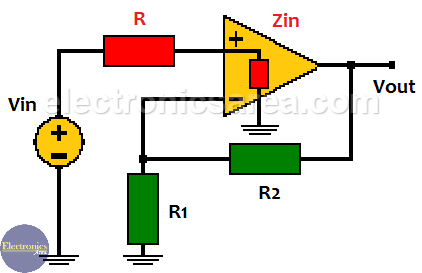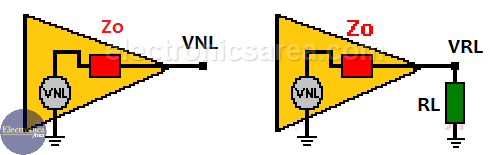# Voltage gain

In a non-inverting operational amplifier (non-inverting amplifier), the signal to be amplified is applied to the non-inverting (+) pin of the integrated circuit. As the name implies, the output signal is not inverted with respect to the input.

The image shows that the voltage at R1 is equal to: VR1 = [R1/(R1 + R2)] x Vout. (voltage divider). In normal operation, the voltage between the inputs (inverter and non-inverter) is practically the same, which means that the Vin input is equal to VR1.

Then if Vin = VR1, and using the above formula: Vin = [R1/(R1 + R2)] x Vout. Clearing for Vout / Vin (voltage / voltage gain). AV = Vout / Vin = (R1 + R2)/R1 = R1/R1 + R2/R1. Then: AV = 1 + R2/R1.

From the above formula it follows that the voltage gain in this type of amplifier will be 1 or greater.## Input impedance

The input impedance of the non-inverting operational amplifier is much greater than that of the inverting amplifier. This value can be obtained experimentally by placing a resistance R of known value at the non-inverting input. Look at the following image.At the terminals of the resistor R there is a voltage drop due to the current flow that comes out of the signal source and enters the operational amplifier.

This current is obtained using ohm’s law: I = VR / R, where VR = Vin – V+. The following formula is used to obtain the input impedance: Zin = V+/I. Where:

• V+: is the voltage at the non-inverting input terminal of the operational amplifier.
• I: is the current previously obtained.

## Output impedance

The output impedance can be obtained (as the input impedance) experimentally.1. The voltage at the output of the operational amplifier without VNL load is measured (If there is no load, there is no current and therefore there is no voltage drop in Zo).
2. A resistor of known value (RL) is placed in the output.
3. Load voltage is measured (nominal voltage) = VRL.
4. The current in the load is obtained using ohm’s law: I = VRL/RL.
5. The output impedance Zo is obtained with the following formula: Zo = [VNL – VRL]/I.

Where:

• Zo = output impedance
• VNL = output voltage of the operational amplifier with no load
• VRL = op amp output voltage with load
•
•
•
•
•
•
•
•
•
•
•
•
•
•
•
•
•
•